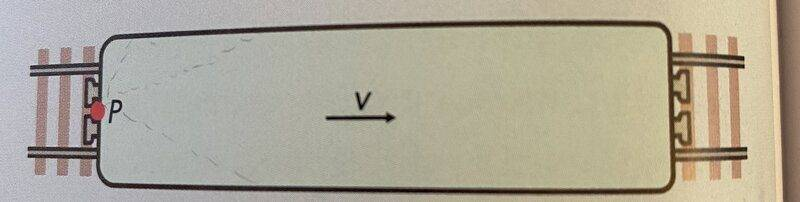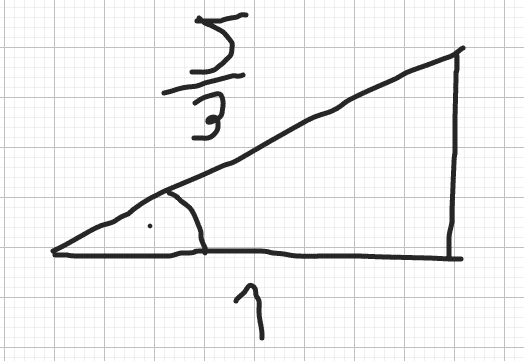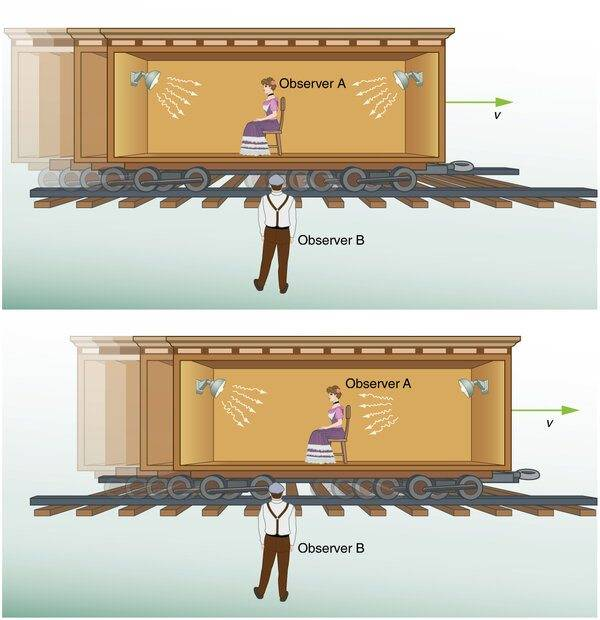# Light beam fired in a moving train (relativity)

• Aristarchus_
In summary, the author does not understand how light could be reflected in multiple directions if the source is not a tilted mirror or another object with specific properties. The author came up with a solution by using the Lorentz factor and using the cosine function.f

#### Aristarchus_

Homework Statement
A train is traveling at a constant velocity v = 0.8c (80% of light speed). The figure shows a train observed from above. A light beam hits point P at the back of the wagon, from where the light is reflected in all directions. There are two questions that need to be answered;
1) I have to explain that some of the light has to be reflected obliquely (tilted, not in a straight line) backward, without hitting the wagon wall.
2) What is the maximal angle that the light beam can be reflected at
Relevant Equations
The formula for length contraction
$$L = \frac{L_0}{\gamma}$$
L_0 is the length of the object observed from the inertial frame of reference, gamma is the Lorentz factor1) really does not make sense to me. It is not clear to me how light could be reflected in multiple directions if the source is not a tilted mirror or another object with specific properties. I think the thought of the "point" P confuses me. Further, the fact that light travels in the opposite direction initially from the velocity vector (direction of the moving train) does not give me any intuition. I suppose it would have to do something with contraction of the train, as I shall attempt in 2)

2) The correct solution that I came up with (which is 53 degrees) was by using the Lorentz factor. Namely, if we calculate

##y = 1/ \sqrt{1-0.8^{2}}##

we obtain a value of y = 5/3. Making a right triangle, We get the value of 53 degrees (I guess the hypothenause would be the refhypotenuseMy question then would be, what and how does this angle of reflection have anything to do with the Lorentz factor and possibly contraction? And if it can be used to potentially answer 1)

I have a rather hostile relationship with LaTex on this site, I will correct it... As ## does not work for me

I have a rather hostile relationship with LaTex on this site, I will correct it... As ## does not work for me
## LaTeX ## "##LaTeX##" puts it inline with text.

$$LaTeX$$ gives its own line

$$LaTeX$$

•Aristarchus_
## LaTeX ## "##LaTeX##" puts it inline with text.

$$LaTeX$$ gives its own line

LaTeXLaTeX​
But '##' does not show symbols for me, so I don't know if it appears for other people then

1) It is not a mirror. It is supposedly a diffuse reflection so the law of reflection does not apply.

2) It is unclear to me how you obtained this answer.

But '##' does not show symbols for me, so I don't know if it appears for other people then
Hit refresh on your browser if it's not showing symbols. Is it still not showing symbols?

•malawi_glenn
2) It is unclear to me how you obtained this answer.
Lorentz factor evaluates to 5/3, and using the ##cos^{-1}(1/\frac{5}{3})## I get the the value of 53 degrees...Now, the question is where is the connection here?

Lorentz factor evaluates to 5/3, and using the ##cos^{-1}(1/\frac{5}{3})## I get the the value of 53 degrees...Now, the question is where is the connection here?
That is what I am asking you. You cannot just throw numerical values around and hope to magically arrive at a solution. You need to make an argument.

•PeroK
That is what I am asking you. You cannot just throw numerical values around and hope to magically arrive at a solution. You need to make an argument.
I understand that I threw numerical values around and got the correct answer. But to rephrase my initial question, so that we do not go in a loop here...Do you have any suggestions as to the connection here?

Question to the Physics Educators:

What is the main point in teaching Special Relativity in high school AP physics?

••PeroK and Aristarchus_
Question to the Physics Educators:

What is the main point in teaching Special Relativity in high school AP physics?
Probably the most mind-boggling chapter I covered in this year's textbook...

Probably the most mind-boggling chapter I covered in this year's textbook...
I'm thinking you could have just barely skimmed the surface by now on physics subjects which you have a chance of making a physical connection to (honing your physics intuition), and here you are seemingly just calculating things. Perhaps there is some reason for teaching it...

•malawi_glenn
What is the main point in teaching Special Relativity in high school AP physics?
To make students aware of it basically. I do not demand them to be able to solve any kind of problem that can not be solved by simple time dilation or length contraction. We also discuss the concept of relativistic total energy which is needed to grasp somewhat before we cover the nuclear physics section of the course.
Probably the most mind-boggling chapter I covered in this year's textbook...
And you are already interested in pursuing theoretical physics? xD Things get waaay more wacky than this!

•erobz and Aristarchus_
To make students aware of it basically. I do not demand them to be able to solve any kind of problem that can not be solved by simple time dilation or length contraction. We also discuss the concept of relativistic total energy which is needed to grasp somewhat before we cover the nuclear physics section of the course.
Yes, it would be very helpful if you could point to the flaws in my problem above, thank you

And you are already interested in pursuing theoretical physics? xD Things get waaay more wacky than this!
I am sure they do :)

Yes, it would be very helpful if you could point to the flaws in my problem above, thank you
Maybe when I get home, I am the gym now (practical physics you know)•erobz and Aristarchus_
Yes, it would be very helpful if you could point to the flaws in my problem above, thank you
I'm not convinced by these AP relativity questions. They seem arbitrary and muddled. My guess for this one is:

In the train frame the light is scattered in all directions to the rear of the ship.

You have to transform that set of light beams to the frame in which the train is moving at ##0.8c## to the right.

•malawi_glenn
In the train frame the light is scattered in all directions to the rear of the ship.
What about the question of being reflected in the tilted direction, without hitting the wagon? How is that possible?

I am having trouble understanding the question still. Some of the wording seems a bit off.
Homework Statement:: A train is traveling at a constant velocity v = 0.8c (80% of light speed). The figure shows a train observed from above. A light beam hits point P at the back of the wagon, from where the light is reflected in all directions. There are two questions that need to be answered;
1) I have to explain that some of the light has to be reflected obliquely (tilted, not in a straight line) backward, without hitting the wagon wall.
I think it should read "hitting the wagon's back wall". A wagon has four walls. Not one.

The word "backward" seems inappropriate. Surely light reflecting from a forward-moving wall can only be reflected "forward".

As I interpret this, we have the light hitting the midpoint P on the train car's back wall.

The light scatters in "all directions". But from the point of view of a passenger in the car, this actually means "in a 180 degree arc". After all, the light cannot scatter through the back wall.

If we are being asked for a scattering angle, we are being asked to consider how the limiting angle (90 degrees on either side of straight forward) changes when we shift to the rest frame of the tracks.

One way to do this would be to write down coordinates of two events in the railroad car frame

1. Reflection event from point P at time zero. (Suggest you place the origin of your coordinate system here).
2. Impact event when reflected light ray hits top of car right at the rear wall.

Repeat for the same two events using the ground frame this time. Tell us what calculations you have used to convert from the "moving" (train car) coordinate system to the "stationary" (train track) system.

Calculate the angle between the two points that you identified in the stationary coordinate system.

•PeroK and malawi_glenn
What about the question of being reflected in the tilted direction, without hitting the wagon? How is that possible?
"Tilted" direction is relative. Even in classical physics that's true.

•jbriggs444
Have you ever been riding a car in the rain? Have you noticed how the rain seems to hit the car at an angle coming from the front and relatively little from the back (or nothing from the back if your back window is vertical enough). This is the same effect as what is considered here, just with rain instead of light.

•jbriggs444 and PeroK
Have you ever been riding a car in the rain? Have you noticed how the rain seems to hit the car at an angle coming from the front and relatively little from the back (or nothing from the back if your back window is vertical enough). This is the same effect as what is considered here, just with rain instead of light.
For some reason, I imagine light traveling always in a straight line. But how does light ever manage not to hit the back wall here while moving in a curved direction, I do not comprehend. The car analogy sounds foreign to me since in this case, light is inside the wagon. That would be like rain inside the car, which would get to the back window if I am understanding your analogy correctly?

For some reason, I imagine light traveling always in a straight line. But how does light ever manage not to hit the back wall here while moving in a curved direction, I do not comprehend. The car analogy sounds foreign to me since in this case, light is inside the wagon. That would be like rain inside the car, which would get to the back window if I am understanding your analogy correctly?
I do not think that you are grasping the analogy correctly. Nothing is curving.

It may be easier to think about light as a stream of machine gun bullets rather than as a straight line. This is important because a beam of light is only a stationary straight line in one frame of reference. In every other frame, a beam of light sweeps across the field of view. Even talking about what angle it has is problematic because it keeps moving while you are trying to measure it.

But it is easier to talk about the angle of the path traced out by a short pulse of light within the beam.

[One could insert some fancy words here about the projection of a trajectory in four dimensional space time onto a three dimensional spatial cross-section of that space time. The direction you choose to make your spatial slices at matters. But you've not been exposed to the geometrical interpretation of Special Relativity yet, so we will not go there]

So you chop out a short segment from the light beam and watch that short segment move. Like a machine gun bullet. Or like a drop of falling rain.

If you are standing still by the side of the road, you see the rain drops falling straight down. But if you are in a moving car, you see them moving diagonally. They are moving horizontally relative to your car as they fall vertically. So naturally they follow the diagonal path. They strike your front windshield. But manage to miss your rear window.

Now consider a short segment of a light ray as it drops from the middle of the train car to the floor. From the train car's point of view it is moving straight down.

But now take the point of view of an observer on the ground. That same segment is being carried along with the train car. So it must be moving diagonally. The ray still moves in a generally downward direction. But it has enough horizontal motion to keep up with the train car as it does so. It falls diagonally in the direction of travel of the train car.

•Aristarchus_Think of two lights that are blinking. Then how each observer will preceive them. Maybe this image will help. You could also think of what you hear when a train goes by at a normal velocity compared to what the passengers hear. Coming toward Observer B the light would be compressed and be shifted to the blue (ultra Violet), traveling away from observer B the light would be extended and shifted toward the red (infrared).

I always think about the EMF of the black body radiation. Do stationary observers see objects coming toward them as hotter and objects moving away as colder? How? Can some one in a liquid swimming pool moving near the speed of light be observed swiming through steam or ice? There is no intuition for realtivity near light speed, just math.

Last edited: